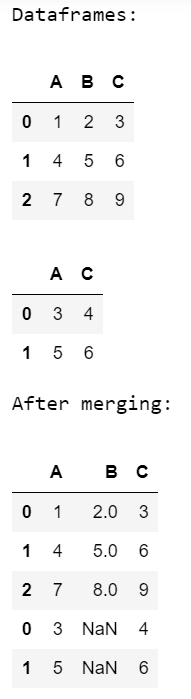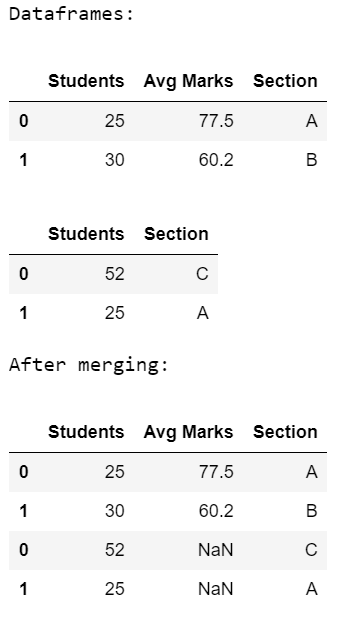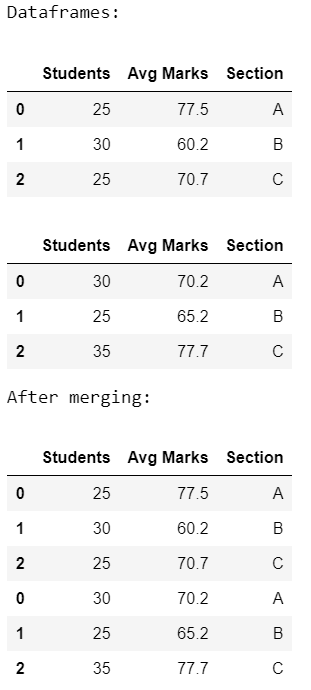# Merge two dataframes with same column names

In order to merge two data frames with the same column names, we are going to use the pandas.concat(). This function does all the heavy lifting of performing concatenation operations along with an axis of Pandas objects while performing optional set logic (union or intersection) of the indexes (if any) on the other axes.

Syntax: concat(objs, axis, join, ignore_index, keys, levels, names, verify_integrity, sort, copy)

### Approach

• Import module
• Create or load first dataframe
• Create or load second dataframe
• Concatenate on the basis of same column names
• Display result

Below are various examples that depict how to merge two data frames with the same column names:

Example 1:

## Python3

 `# import module ` `import` `pandas as pd ` ` `  `# assign dataframes ` `data1 ``=` `pd.DataFrame([[``1``, ``2``, ``3``], [``4``, ``5``, ``6``], [``7``, ``8``, ``9``]], ` `                     ``columns``=``[``'A'``, ``'B'``, ``'C'``]) ` ` `  `data2 ``=` `pd.DataFrame([[``3``, ``4``], [``5``, ``6``]], ` `                     ``columns``=``[``'A'``, ``'C'``]) ` ` `  `# display dataframes ` `print``(``'Dataframes:'``) ` `display(data1) ` `display(data2) ` ` `  `# merge two data frames ` `print``(``'After merging:'``) ` `pd.concat([data1, data2], axis``=``0``) `

Output:Example 2:

## Python3

 `# import module ` `import` `pandas as pd ` ` `  `# assign dataframes ` `data1 ``=` `pd.DataFrame([[``25``, ``77.5``, ``'A'``], [``30``, ``60.2``, ``'B'``]], ` `                     ``columns``=``[``'Students'``, ``'Avg Marks'``, ``'Section'``]) ` ` `  `data2 ``=` `pd.DataFrame([[``52``, ``'C'``], [``25``, ``'A'``]], ` `                     ``columns``=``[``'Students'``, ``'Section'``]) ` ` `  `# display dataframes ` `print``(``'Dataframes:'``) ` `display(data1) ` `display(data2) ` ` `  `# merge two data frames ` `print``(``'After merging:'``) ` `pd.concat([data1, data2], axis``=``0``) `

Output:Example 3:

## Python3

 `# import module ` `import` `pandas as pd ` ` `  `# assign dataframes ` `data1 ``=` `pd.DataFrame([[``25``, ``77.5``, ``'A'``], [``30``, ``60.2``, ``'B'``], ` `                      ``[``25``, ``70.7``, ``'C'``]], ` `                     ``columns``=``[``'Students'``, ``'Avg Marks'``, ``'Section'``]) ` ` `  `data2 ``=` `pd.DataFrame([[``30``, ``70.2``, ``'A'``], [``25``, ``65.2``, ``'B'``], ` `                      ``[``35``, ``77.7``, ``'C'``]], ` `                     ``columns``=``[``'Students'``, ``'Avg Marks'``, ``'Section'``]) ` ` `  ` `  `# display dataframes ` `print``(``'Dataframes:'``) ` `display(data1) ` `display(data2) ` ` `  `# merge two data frames ` `print``(``'After merging:'``) ` `pd.concat([data1, data2], axis``=``0``) `

Output:Whether you're preparing for your first job interview or aiming to upskill in this ever-evolving tech landscape, GeeksforGeeks Courses are your key to success. We provide top-quality content at affordable prices, all geared towards accelerating your growth in a time-bound manner. Join the millions we've already empowered, and we're here to do the same for you. Don't miss out - check it out now!

Previous
Next